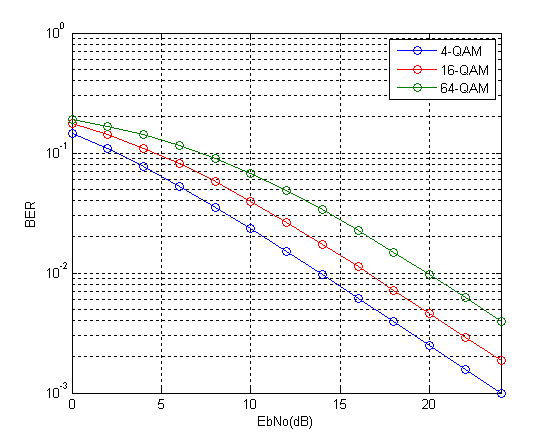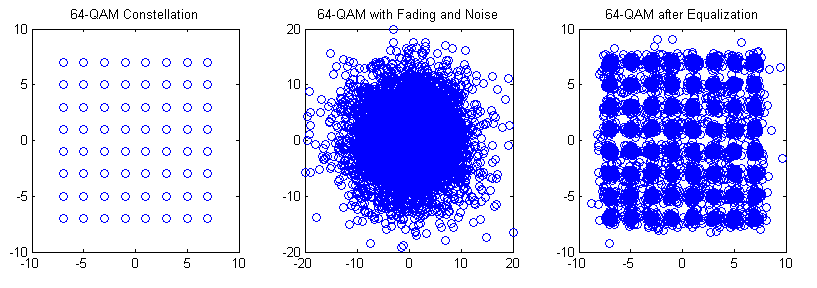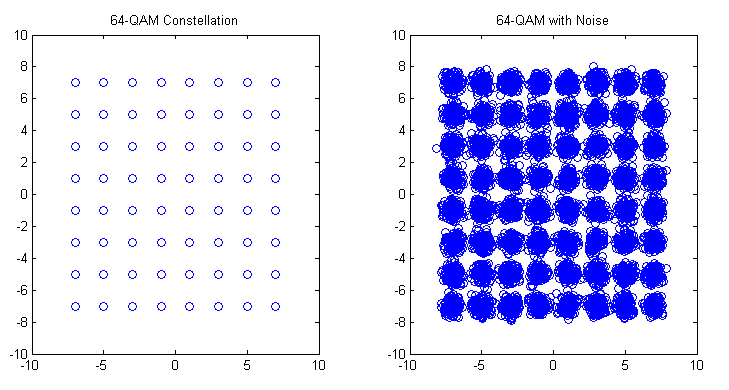# Theoretical BER of M-QAM in Rayleigh Fading

We have previously discussed the Bit Error Rate of M-QAM in Rayleigh Fading using Monte Carlo Simulation. We now turn our attention to calculation of Bit Error Rate (BER) of M-QAM in Rayleigh fading using analytical techniques. In particular we look at the method used in MATLAB function berfading.m. In this function the BER of 4-QAM, 16-QAM and 64-QAM is calculated from series expressions having 1, 3 and 5 terms respectively. These are given below (M is the constellation size and must be a power of 2).

```if (M == 4)
ber = 1/2 * ( 1 - sqrt(gamma_c/k./(1+gamma_c/k)) );
elseif (M == 16)
ber = 3/8 * ( 1 - sqrt(2/5*gamma_c/k./(1+2/5*gamma_c/k)) ) ...
+ 1/4 * ( 1 - sqrt(18/5*gamma_c/k./(1+18/5*gamma_c/k)) ) ...
- 1/8 * ( 1 - sqrt(10*gamma_c/k./(1+10*gamma_c/k)) );
elseif (M == 64)
ber = 7/24 * ( 1 - sqrt(1/7*gamma_c/k./(1+1/7*gamma_c/k)) ) ...
+ 1/4 * ( 1 - sqrt(9/7*gamma_c/k./(1+9/7*gamma_c/k)) ) ...
- 1/24 * ( 1 - sqrt(25/7*gamma_c/k./(1+25/7*gamma_c/k)) ) ...
+ 1/24 * ( 1 - sqrt(81/7*gamma_c/k./(1+81/7*gamma_c/k)) ) ...
- 1/24 * ( 1 - sqrt(169/7*gamma_c/k./(1+169/7*gamma_c/k)) );
```

Although using these expressions we get very accurate BER but it is not that simple to calculate (the expressions become even more complicated for higher constellation sizes such as 256-QAM). Therefore we try to simplify these expressions by using only the first term in each expression. To our surprise the results match quite well with the results using the exact formulae. There is very minor difference at low signal to noise ratios but that can be easily bargained for the ease of calculation.

So here is our program for calculating the BER using the approximate method.

```%%%%%%%%%%%%%%%%%%%%%%%%%%%%%%%%%%%%%%%%%%%%%%%%%%%%%%%%%%%%%%%%%%%%%%%%%%%
% FUNCTION TO CALCULATE THE BER OF M-QAM IN RAYLEIGH FADING
% M: Input, Constellation Size
% EbNo: Input, Energy Per Bit to Noise Power Spectral Density
% ber: Output, Bit Error Rate
%%%%%%%%%%%%%%%%%%%%%%%%%%%%%%%%%%%%%%%%%%%%%%%%%%%%%%%%%%%%%%%%%%%%%%%%%%%

k=log2(M);
EbNoLin=10.^(EbNo/10);
gamma_c=EbNoLin*k;

if M==4
%4-QAM
ber = 1/2 * ( 1 - sqrt(gamma_c/k./(1+gamma_c/k)) );
elseif M==16
%16-QAM
ber = 3/8 * ( 1 - sqrt(2/5*gamma_c/k./(1+2/5*gamma_c/k)) );
elseif M==64
%64-QAM
ber = 7/24 * ( 1 - sqrt(1/7*gamma_c/k./(1+1/7*gamma_c/k)) );
else
%Warning
warning('M=4,16,64')
ber=zeros(1,length(EbNo));
end

semilogy(EbNo,ber,'o-')
xlabel('EbNo(dB)')
ylabel('BER')
axis([0 24 0.001 1])
grid on

return
%%%%%%%%%%%%%%%%%%%%%%%%%%%%%%%%%%%%%%%%%%%%%%%%%%%%%%%%%%%%%%%%%%%%%%%%%%%
```So we see that the results match quite well with the results previously obtained through simulation. We will next tackle the problem of simplifying the expression for higher order modulations such as 256-QAM in both Rayleigh and Ricean channels.

# M-QAM Bit Error Rate in Rayleigh Fading

We have previously discussed the bit error rate (BER) performance of M-QAM in AWGN. We now discuss the BER performance of M-QAM in Rayleigh fading. The one-tap Rayleigh fading channel is generated from two orthogonal Gaussian random variables with variance of 0.5 each. The complex random channel coefficient so generated has an amplitude which is Rayleigh distributed and a phase which is uniformly distributed. As usual the fading channel introduces a multiplicative effect whereas the AWGN is additive.

The function “QAM_fading” has three inputs, ‘n_bits’, ‘M’, ‘EbNodB’ and one output ‘ber’. The inputs are the number of bits to be passed through the channel, the alphabet size and the Energy per Bit to Noise Power Spectral Density in dB respectively whereas the output is the bit error rate (BER).

```%%%%%%%%%%%%%%%%%%%%%%%%%%%%%%%%%%%%%%%%%%%%%%%%%%%%%%%%%%%%%%%%%%
% FUNCTION THAT CALCULATES THE BER OF M-QAM IN RAYLEIGH FADING
% n_bits: Input, number of bits
% M: Input, constellation size
% EbNodB: Input, energy per bit to noise power spectral density
% ber: Output, bit error rate
%%%%%%%%%%%%%%%%%%%%%%%%%%%%%%%%%%%%%%%%%%%%%%%%%%%%%%%%%%%%%%%%%%
% Transmitter
k=log2(M);
EbNo=10^(EbNodB/10);
x=transpose(round(rand(1,n_bits)));
h1=modem.qammod(M);
h1.inputtype='bit';
h1.symbolorder='gray';
y=modulate(h1,x);

% Channel
Eb=mean((abs(y)).^2)/k;
sigma=sqrt(Eb/(2*EbNo));
w=sigma*(randn(n_bits/k,1)+1i*randn(n_bits/k,1));
h=(1/sqrt(2))*(randn(n_bits/k,1)+1i*randn(n_bits/k,1));
r=h.*y+w;

r=r./h;
h2=modem.qamdemod(M);
h2.outputtype='bit';
h2.symbolorder='gray';
h2.decisiontype='hard decision';
z=demodulate(h2,r);
ber=(n_bits-sum(x==z))/n_bits
return
%%%%%%%%%%%%%%%%%%%%%%%%%%%%%%%%%%%%%%%%%%%%%%%%%%%%%%%%%%%%%%%%%%
```The bit error rates of four modulation schemes 4-QAM, 16-QAM, 64-QAM and 256-QAM are shown in the figure above. All modulation schemes use Gray coding which gives a few dB of margin in the BER performance. As with the AWGN case each additional bit per symbol requires about 1.5-2 dB in signal to ratio to achieve the same BER.

Although not shown here similar behavior is observed for higher order modulation schemes such as 1024-QAM and 4096-QAM (the gap in the signal to noise ratio for the same BER is increased to about 5dB).

Lastly we explain some of the terms used above.

Rayleigh Fading is a commonly used term in simulation of Digital Communication Systems but it tends to differ in meaning in different contexts. The term Rayleigh Fading as used above means a single tap channel that varies from one symbol to the next. It has an amplitude which is Rayleigh distributed and a phase which is Uniformly distributed. A single tap channel means that it does not introduce any Inter Symbol Interference (ISI). Such a channel is also referred to as a Flat Fading Channel. The channel can also be referred to as a Fast Fading Channel since each symbol experiences a new channel state which is independent of its previous state (also termed as uncorrelated).

Gray Coding

When using QAM modulation, each QAM symbol represents 2,3,4 or higher number of bits. That means that when a symbol error occurs a number of bits are reversed. Now a good way to do the bit-to-symbol assignment is to do it in a way such that no neighboring symbols differ by more than one bit e.g. in 16-QAM, a symbol that represents a binary word 1101 is surrounded by four symbols representing 0101, 1100, 1001 and 1111. So if a symbol error is made, only one bit would be in error. However, one must note that this is true only in good signal conditions. When the SNR is low (noise has a higher magnitude) the symbol might be displaced to a location that is not adjacent and we might get higher number of bits in error.

Hard Decision

The concept of hard decision decoding is important when talking about channel coding, which we have not used in the above simulation. However, we will briefly explain it here. Hard decision is based on what is called “Hamming Distance” whereas soft decision is based on what it called “Euclidean Distance”. Hamming Distance is the distance of a code word in binary form, such as 011 differs from 010 and 001 by 1. Whereas the Euclidean distance is the distance before a decision is made that a bit is zero or one.  So if the received sequence is 0.1 0.6 0.7 we get a Euclidean distance of 0.8124 from 010 and 0.6782 from 001. So we cannot make a hard decision about which sequence was transmitted based on the received sequence of 011. But based on the soft metrics we can make a decision that 001 was the most likely sequence that was transmitted (assuming that 010 and 001 were the only possible transmitted sequences).

# M-QAM Bit Error Rate in AWGN

Quadrature Amplitude Modulation has been adopted by most wireless communication standards such as WiMAX and LTE. It provides higher bit rates and consequently higher spectral efficiencies. It is usually used in conjunction with Orthogonal Frequency Division Multiplexing (OFDM) which provides a simple technique to overcome the time varying frequency selective channel.

We have previously discussed the formula for calculating the bit error rate (BER) of QAM in AWGN. We now calculate the same using a simple Monte Carlo Simulation.

```%%%%%%%%%%%%%%%%%%%%%%%%%%%%%%%%%%%%%%%%%%%%%%%%%%%%%%%%%%%%%%%%%%
% FUNCTION THAT CALCULATES THE BER OF M-QAM IN AWGN
% n_bits: Input, number of bits
% M: Input, constellation size
% EbNodB: Input, energy per bit to noise power spectral density
% ber: Output, bit error rate
%%%%%%%%%%%%%%%%%%%%%%%%%%%%%%%%%%%%%%%%%%%%%%%%%%%%%%%%%%%%%%%%%%

function[ber]= QAM_AWGN(n_bits, M, EbNodB)

% Transmitter
k=log2(M);
EbNo=10^(EbNodB/10);
x=transpose(round(rand(1,n_bits)));
h1=modem.qammod(M);
h1.inputtype='bit';
h1.symbolorder='gray';
y=modulate(h1,x);

% Channel
Eb=mean((abs(y)).^2)/k;
sigma=sqrt(Eb/(2*EbNo));
w=sigma*(randn(1,n_bits/k)+1i*randn(1,n_bits/k));
r=y+w';

h2=modem.qamdemod(M);
h2.outputtype='bit';
h2.symbolorder='gray';
h2.decisiontype='hard decision';
z=demodulate(h2,r);
ber=(n_bits-sum(x==z))/n_bits
return
%%%%%%%%%%%%%%%%%%%%%%%%%%%%%%%%%%%%%%%%%%%%%%%%%%%%%%%%%%%%%%%%%%
```The above function basically has three inputs and one output. The inputs are the number of bits to be passed through the channel, the size of the constellation and the signal to noise ratio in dB. The output is the bit error rate (BER). The simulation can be divided into three section namely the transmitter, the channel and the receiver. In this simulation we have used Gray coding which gives us about a dB of improvement at low to medium signal to noise ratio.

As seen above the BER obtained through our simulation matches quite well with the BER obtained through the theoretical formula. Each additional bit per symbol required about 2dB extra in signal to noise ratio to achieve the same bit error rate.

Lastly we explain some of the terms used above.

AWGN

All wireless receivers suffer from thermal noise which is a function of absolute temperature and bandwidth of the receiver. This noise is added to the received signal and makes detection of weak signals a major challenge. Just to given you an idea typical GSM receivers have a noise floor of -113 dBm. Therefore, if the received signal has a power of -100 dBm we get a signal to noise ratio (SNR) of 13 dB. In simulation this noise is usually modeled as a Gaussian Random Process. It is additive, as opposed to channel impairments which are multiplicative and has a flat spectrum (thus called White Noise).

Gray Coding

When using QAM modulation, each QAM symbol represents 2,3,4 or higher number of bits. That means that when a symbol error occurs a number of bits are reversed. Now a good way to do the bit-to-symbol assignment is to do it in a way such that no neighboring symbols differ by more than one bit e.g. in 16-QAM, a symbol that represents a binary word 1101 is surrounded by four symbols representing 0101, 1100, 1001 and 1111. So if a symbol error is made, only one bit would be in error. However, one must note that this is true only in good signal conditions. When the SNR is low (noise has a higher magnitude) the symbol might be displaced to a location that is not adjacent and we might get higher number of bits in error.

Hard Decision

The concept of hard decision decoding is important when talking about channel coding, which we have not used in the above simulation. However, we will briefly explain it here. Hard decision is based on what is called “Hamming Distance” whereas soft decision is based on what it called “Euclidean Distance”. Hamming Distance is the distance of a code word in binary form, such as 011 differs from 010 and 001 by 1. Whereas the Euclidean distance is the distance before a decision is made that a bit is zero or one.  So if the received sequence is 0.1 0.6 0.7 we get a Euclidean distance of 0.8124 from 010 and 0.6782 from 001. So we cannot make a hard decision about which sequence was transmitted based on the received sequence of 011. But based on the soft metrics we can make a decision that 001 was the most likely sequence that was transmitted (assuming that 010 and 001 were the only possible transmitted sequences).

# QAM Theoretical BER in AWGN

Quadrature Amplitude Modulation (QAM) is an important modulation scheme as it allows for higher data rates and spectral efficiencies. The bit error rate (BER) of QAM can be calculated through Monte Carlo simulations. However this becomes quite complex as the constellation size of the modulation schemes increases. Therefore a theoretical approach is sometimes preferred. The BER for Gray coded QAM, for even number of bits per symbol, is shown below.

Gray coding ensures that a symbol error results in a single bit error. The code for calculating the theoretical QAM BER for k even (even number of bits per symbol) is given below. The formula for calculating the BER for k odd is different, however, the formula given below can be used a first estimate.

```EbNodB=-6:2:24
EbNo=10.^(EbNodB/10);
k=8;
M=2^k;
x=sqrt(3*k*EbNo/(M-1));
Pb=(4/k)*(1-1/sqrt(M))*(1/2)*erfc(x/sqrt(2));
semilogy(EbNodB,Pb)```

Note:
1. Each additional bit/symbol requires about 2dB extra in SNR to achieve the same BER.
2. 4-QAM is essentially QPSK modulation.

# BER of 64-QAM OFDM in Frequency Selective Fading-II

In the previous post we had considered a static frequency-selective channel. We now consider a time-varying frequency selective channel with 7 taps. Each tap of the time domain filter has a Gaussian distributed real component with variance 1/(2*n_tap) and a Gaussian distributed imaginary component with variance 1/(2*n_tap). The amplitude of each tap is thus Rayleigh distributed and the phase is Uniformly distributed. Since the power in each component is normalized by the filter length (n_tap) the BER performance would remain the same even if the filter length is changed (this has been verified experimentally).

```%%%%%%%%%%%%%%%%%%%%%%%%%%%%%%%%%%%%%%%%%%%%%%%%%%%%%%%%%%%%%%%%%%%%%%%%%
% FUNCTION TO SIMULATE PERFORMANCE OF 64-OFDM IN TIME VARYING FREQUENCY SELECTIVE CHANNEL
% n_bits: Input, length of binary sequence
% n_fft: Input, length of FFT (Fast Fourier Transform)
% EbNodB: Input, energy per bit to noise power spectral density ratio
% ber: Output, bit error rate
%%%%%%%%%%%%%%%%%%%%%%%%%%%%%%%%%%%%%%%%%%%%%%%%%%%%%%%%%%%%%%%%%%%%%%%%%

Eb=7;
M=64;
k=log2(M);
n_cyc=32;
EbNo=10^(EbNodB/10);
x=transpose(round(rand(1,n_bits)));
h1=modem.qammod(M);
h1.inputtype='bit';
h1.symbolorder='gray';
y=modulate(h1,x);
n_sym=length(y)/n_fft;
n_tap=7;

for n=1:n_sym;
s_ofdm=sqrt(n_fft)*ifft(y((n-1)*n_fft+1:n*n_fft),n_fft);
s_ofdm_cyc=[s_ofdm(n_fft-n_cyc+1:n_fft); s_ofdm];
ht=(1/sqrt(2))*(1/sqrt(n_tap))*(randn(1,n_tap)+j*randn(1,n_tap));
Hf=fft(ht,n_fft);
r_ofdm_cyc=conv(s_ofdm_cyc,ht);
r_ofdm_cyc=(r_ofdm_cyc(1:n_fft+n_cyc));
wn=sqrt((n_fft+n_cyc)/n_fft)*(randn(1,n_fft+n_cyc)+j*randn(1,n_fft+n_cyc));
r_ofdm_cyc=r_ofdm_cyc+sqrt(Eb/(2*EbNo))*wn.';
r_ofdm=r_ofdm_cyc(n_cyc+1:n_fft+n_cyc);
s_est((n-1)*n_fft+1:n*n_fft)=(fft(r_ofdm,n_fft)/sqrt(n_fft))./Hf.';
end

h2=modem.qamdemod(M);
h2.outputtype='bit';
h2.symbolorder='gray';
h2.decisiontype='hard decision';
z=demodulate(h2,s_est.');
ber=(n_bits-sum(x==z))/n_bits
return
%%%%%%%%%%%%%%%%%%%%%%%%%%%%%%%%%%%%%%%%%%%%%%%%%%%%%%%%%%%%%%%%%%%%%%%%%
```

As before we have used an FFT size of 128 and cyclic prefix of 32 samples. The FFT and IIFT operations are normalized to maintain the signal to noise ratio (SNR). The extra energy transmitted in the cyclic prefix is also accounted for in the SNR calibration.

It is observed that the BER performance of 64-QAM OFDM in the time-varying frequency-selective channel is quite similar to that in the static frequency-selective channel with complex filter taps. It must be noted that with 64-QAM the goal is to achieve higher bit rate, error rates can be improved using antenna diversity and channel coding schemes.

Given below is the wrapper that should be used along with the above code. The wrapper basically calls the above routine for each value of EbNodB. The length of the binary sequence and the FFT size are other inputs to the function. The bit error rate at the specific EbNodB is the output of the function.

```%%%%%%%%%%%%%%%%%%%%%%%%%%%%%%%%%%%
clear all;
close all;
k=6;
n_fft=128;
l=k*n_fft*1e3;
EbNodB=0:2:20;
for n=1:length(EbNodB);n
end;
semilogy(EbNodB,ber,'O-');
grid on
xlabel('EbNo')
ylabel('BER')
%%%%%%%%%%%%%%%%%%%%%%%%%%%%%%%%%%%```

In future we would use the standard LTE channel models, namely EPA, EVA and ETU in our simulation.

# LTE Data Rate Calculation

Peak LTE data rate can be calculated using the following parameters:

1 Time-slot=0.5 ms (i.e 1 Sub-frame = 1 ms)
1 Time-slot=7 Modulation Symbols (when normal CP length is used)
1 Modulation Symbol=6 bits; if 64 QAM is used as modulation scheme

Data rate for a single carrier=Number of symbols per time slot*Bits per symbol/Duration of a time slot=7*6/0.5e-3=84kbps

If 1200 carriers (100RBs) are used then the aggregated throughput would be=1200*84kbps=100.8Mbps

If 4×4 MIMO is used then the capacity would increase four fold to=403.2Mbps

With 3/4 channel coding the data rate would be reduced to=302.4Mbps

Note:

1. A Resource Block (RB)=12 Carriers

2. Actual data rate would depend upon the instantaneous channel conditions and number of users sharing the resources e.g. going down from 64QAM to QPSK in adverse channel conditions would reduce the data rate from 302.4Mbps to 100.8Mbps.  Changing the code rate from 3/4 to 1/3 would further reduce the data rate to 44.8Mbps.